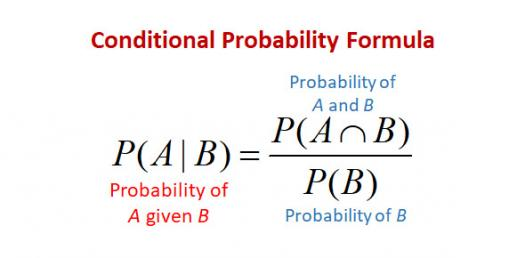# Conditional Probability Quiz

12 Questions | Attempts: 1331
ShareSettingsThis is a self-check quiz on probability. Take this quiz and see how well do you know about it!

• 1.
The letters of the name SMISS are arranged in a line. If an arrangement is chosen at random, what is the probability that the three Ss are together?
• A.

0.6

• B.

0.3

• C.

0.1

• D.

0.4

• 2.
A box contains 36 marbles. If a marble is picked at random, the probability of being red is 2/9. How many red marbles should be added to make this probability 1/3?
• A.

6

• B.

4

• C.

12

• D.

9

• 3.
An identity card is picked at random. What is the probability that the sum of the last two digits of its number is 9?
• A.

0.5

• B.

0.4

• C.

0.1

• D.

0.2

• 4.
One of the 5 points (3, 2), (2, 1), (1, - 4), (5, 5) and (4, 6) is selected at random. What is the probability that it lies on the straight line 3x – 2y = 5?
• A.

0.4

• B.

0.2

• C.

0.1

• D.

0.5

• 5.
The letters of the word “PROBABILITY” are written on cards and the cards are then shuffled. If a card is picked at random, find the probability that it will contain a vowel.
• A.

5/11

• B.

3/11

• C.

7/11

• D.

4/11

• 6.
I have two 10-cent coins, three 20-cent coins, four 50-cent coins and five \$1 coins in my pocket. If I choose a coin at random, find the probability that the coin is worth at least 50 cents.
• A.

4/7

• B.

3/7

• C.

9/14

• D.

5/14

• 7.
A man tosses two fair dice. One is numbered 1 to 6 in the usual way and the other is numbered 1, 3, 5, 7, 9 and 11. Find the probability that the total of the two numbers shown is greater than 10.
• A.

7/12

• B.

5/12

• C.

1/2

• D.

1/4

• 8.
A card is drawn at random from a normal pack of 52 cards. If A represents the event that the card drawn is a Queen and B represents the event that the card drawn is a Heart. Find P(A|B).
• A.

5/13

• B.

1/4

• C.

4/13

• D.

1/52

• 9.
Two fair dice, one red and one blue, are tossed. What is the probability that the total of the numbers shown by the two dice exceeds 3?
• A.

1/3

• B.

1/4

• C.

11/12

• D.

1/12

• 10.
A bag contains 30 balls. The balls are numbered 1,2,3,4 ….30. A ball is drawn at random. Find the probability that the number on the ball is a prime number.
• A.

8/9

• B.

2/3

• C.

1/3

• D.

4/9

• 11.
An interview with 18 people revealed that 5 of the 8 women and 8 of the 10 men preferred drinking coffee to tea. What is the probability that if one person is selected from the group of 18 people, it is either a woman or someone who preferred to drink coffee than tea?
• A.

8/9

• B.

1/3

• C.

1/9

• D.

2/3

• 12.
A computer generates 4-digit random numbers in the range 0000 to 9999 inclusive. Find the probability that the computer produces a random number that begins and ends with the digit 1.
• A.

1/10

• B.

1/4

• C.

1/1000

• D.

1/100

## Related TopicsBack to top
×

Wait!
Here's an interesting quiz for you.